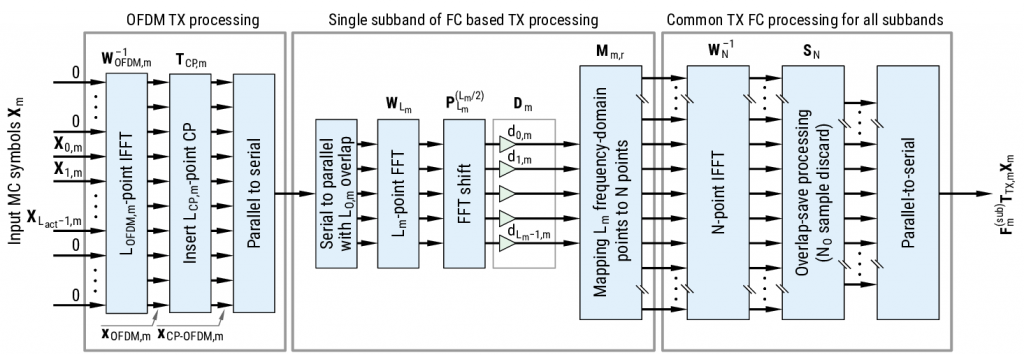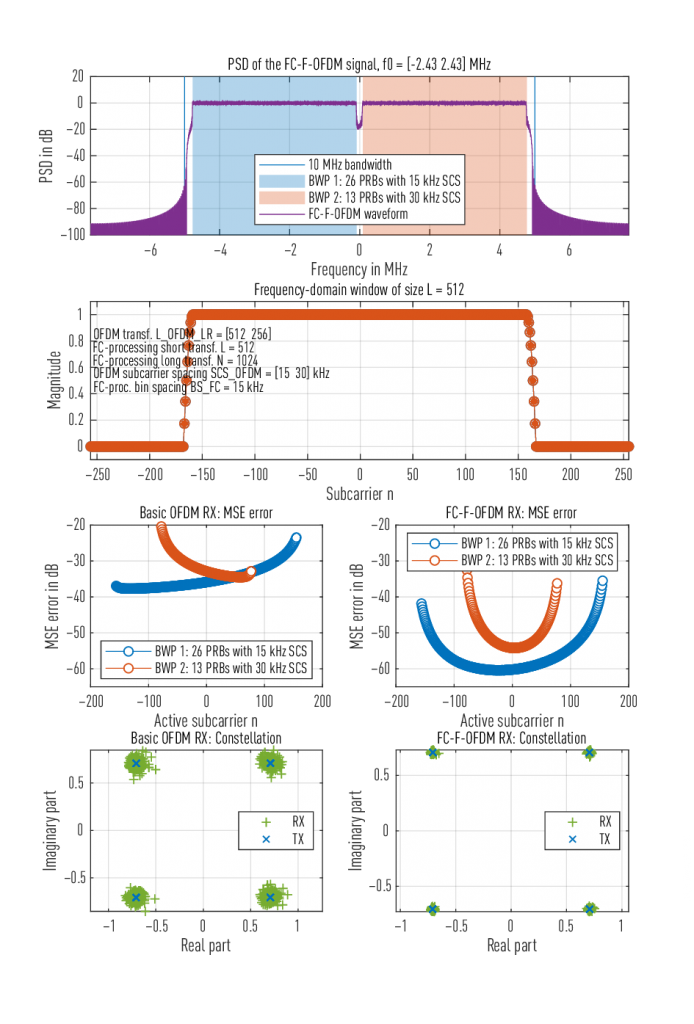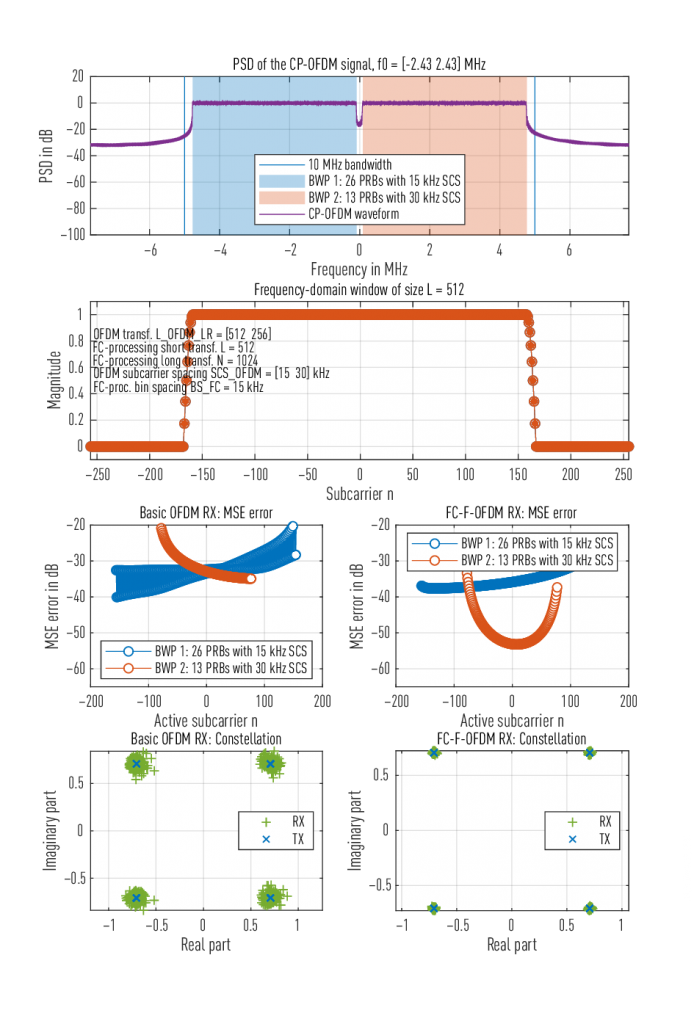# Efficient fast-convolution based waveform processing for 5G physical layer

Page

Juha Yli-Kaakinen, Toni Levanen, Sami Valkonen, Kari Pajukoski, Juho Pirskanen, Markku Renfors, and Mikko Valkama, “Efficient fast-convolution based waveform processing for 5G physical layer,” IEEE . Select. Areas in Commun., (Special Issue on Deployment Issues and Performance Challenges for 5G), vol. 35, no. 6, pp. 1309–1326, 2017. DOI: 10.1109/JSAC.2017.2687358 (arXiv:1706.02853)

## Abstract

This paper investigates the application of fast-convolution (FC) filtering schemes for flexible and effective waveform generation and processing in the fifth generation (5G) systems. FC-based filtering is presented as a generic multimode waveform processing engine while, following the progress of 5G new radio standardization in the Third-Generation Partnership Project, the main focus is on efficient generation and processing of subband-filtered cyclic prefix orthogonal frequency-division multiplexing (CP-OFDM) signals. First, a matrix model for analyzing FC filter processing responses is presented and used for designing optimized multiplexing of filtered groups of CP-OFDM physical resource blocks (PRBs) in a spectrally well-localized manner, i.e., with narrow guardbands. Subband filtering is able to suppress interference leakage between adjacent subbands, thus supporting independent waveform parametrization and different numerologies for different groups of PRBs, as well as asynchronous multiuser operation in uplink. These are central ingredients in the 5G waveform developments, particularly at sub-6-GHz bands. The FC filter optimization criterion is passband error vector magnitude minimization subject to a given subband band-limitation constraint. Optimized designs with different guardband widths, PRB group sizes, and essential design parameters are compared in terms of interference levels and implementation complexity. Finally, extensive coded 5G radio link simulation results are presented to compare the proposed approach with other subband-filtered CP-OFDM schemes and time-domain windowing methods, considering cases with different numerologies or asynchronous transmissions in adjacent subbands. Also the feasibility of using independent transmitter and receiver processing for CP-OFDM spectrum control is demonstrated.Figure 1: FC-F-OFDM transmitter. The processing structure for one filtered group of PRBs is shown. The long N-point IFFT is common for all the subbands.

## Matlab/Octave model

Simple Matlab/Octave model for illustrating the fast-convolution (FC)-based filtered OFDM (FC-F-OFDM) processing is given below. In this model, composite waveform containing two bandwidth parts (BWPs) with 15 kHz and 30 kHz subcarrier spacing (SCS) is generated and this waveform is received using plain CP-OFDM RX and FC-based RX. For 15 kHz SCS, 26 physical resourse blocks (PRBs) are active whereas, for 30 kHz SCS, 12 PRBs are active. The guardband between BWPs is 180 kHz.

Without input arguments or when called with `FC_F_OFDM("FC-F-OFDM")`, this model uses FC-based synthesis filterbank for generating the TX waveform. When called with `FC_F_OFDM("CP-OFDM")`, simulation results with plain CP-OFDM TX are shown. These results for FC-F-OFDM and CP-OFDM TX are shown below in Figs. 2 and 3, respectively. As seen from these figures, the out-of-band (OOB) emissions for the FC-F-OFDM TX are considerably lower (well below −60 dB) when compared to plain CP-OFDM TX (only −21 dB). In addition, the performance of the FC-F-OFDM RX in terms of in-band MSE is better for both TX alternatives.

```%% Description: Model for illustrating the channelization of two 5 MHz bandwidth parts
%%              within a 10 MHz channel using the fast-convolution-based filterbank.
%%
%% Author: Juha Yli-Kaakinen (juha.yli-kaakinen@tuni.fi),
%%         Tampere University, Finland
%% Created: Mon Jan 22 13:11:42 2018
%% Version: \$1.0\$
%% Last-Updated: Fri Nov  8 13:24:10 2019
%%           By: Juha Yli-Kaakinen
%%     Update #: 3127
%% Keywords:
%% References:
%%  Markku Renfors, Juha Yli-Kaakinen, Toni Levanen, Mikko Valkama, Tero Ihalainen,
%%     and Jaakko Vihriala "Efficient fast-convolution implementation of filtered
%%     CP-OFDM waveform processing for 5G," in Proc. IEEE GC 2015 Workshop on 5G &
%%     Beyond - Enabling Technologies and Applications (in conjunction with IEEE
%%     GLOBECOM), San Diego, CA, USA, Dec 6-10, 2015.
%%     https://doi.org/10.1109/GLOCOMW.2015.7414034
%%  Juha Yli-Kaakinen, Toni Levanen, Sami Valkonen, Kari Pajukoski, Juho Pirskanen,
%%     Markku Renfors, and Mikko Valkama, "Efficient fast-convolution based waveform
%%     processing for 5G physical layer," IEEE Journal on Selected Areas in
%%     Communications (Special Issue on Deployment Issues and Performance
%%     Challenges for 5G), vol. 35, no. 6, pp. 1309-1326, 2017.
%%     https://doi.org/10.1109/JSAC.2017.2687358
%%%%%%%%%%%%%%%%%%%%%%%%%%%%%%%%%%%%%%%%%%%%%%%%%%%%%%%%%%%%%%%%%%%%%%%%%%%%%%%%%%%%%%
function FC_F_OFDM(TXproc)

if (nargin == 0)
TXproc = 'FC-F-OFDM';

end

ran_seed = 10;

isOctave = exist('OCTAVE_VERSION', 'builtin') == 5;
if isOctave
randn('seed', ran_seed);
else
rng(ran_seed);
end

vec = @(x) x(:);            % Vectorization function

nAct = [26; 13]*12;         % Number of active subcarriers (25 and 11 physical
%   resource blocks (PRBs) for 1st and 2nd bandwidth
%   part (BWP), respectively)
L = 512;                    % Fast-convolution (FC) processing short transform (FFT)
%   size (L_m in )
I = 2;                      % Interpolation factor
N = I*L;                    % FC processing long transform (IFFT) size
L_OFDM_LR = [512; 256];     % TX OFDM IFFT sizes for BWPs giving 15 kHz and 30 kHz
%   subcarrier spacings (SCSs)
Bin0 = 162*[-1; 1];         % Center bins of the BWPs (180 kHz guardband)

Fs = N/L*L_OFDM_LR(1)*15e3; % Output sampling rate (30.72 MHz)

bOFDM = [14; 28];           % Number of OFDM symbols (14 for 15 kHz SCS and 28 for
%   30 kHz SCS)
L_OFDM_HR = I*L_OFDM_LR;    % OFDM FFT size on the high-rate side

BS_FC = Fs/N;               % FC processing bin spacing (BS)
SCS_OFDM = Fs/I./L_OFDM_LR; % OFDM processing SCS
SCS_fac = SCS_OFDM/BS_FC;   % Ratio between OFDM SCS and FC processing SCS

L_CP_LR = 9*L_OFDM_LR/128;  % CP length on the low-rate side

% Symbol-wise CP lengths on the low-rate side
L_CP_LR(1, 1:bOFDM(1)) = 9*L_OFDM_LR(1)/128;     % CP length for BWP 1
L_CP_LR(1, 1:7:end)  = L_CP_LR(1, 1:7:end) + 8;  % For the 1st symbols in a slot
L_CP_LR(2, 1:bOFDM(2)) = 9*L_OFDM_LR(2)/128;     % CP length for BWP 2
L_CP_LR(2, 1:14:end) = L_CP_LR(2, 1:14:end) + 8; % For the 1st symbols in a slot

L_CP_HR = I*L_CP_LR;        % CP length on the high-rate side

nTB = 8;                    % Number of transition band weights
excessBW = 3;               % Excess bandwidth in terms of FC bins

assert(all(nAct < L_OFDM_LR), ...
'Number active subcarriers has to smaller than OFDM transform size')
assert(all(nAct.*SCS_fac+2*nTB < L), ...
['Number of non-zero bins of the FD window has to be smaller than ' ...
'FC processing short transform'])

OLF = 1/2;                  % Overlap factor
Ls = round(L*(1 - OLF));    % Non-overlapping part on the low-rate side
Ll = ceil((L - Ls)/2);      % Leading overlapping part on the low-rate side
Ns = round(N*(1 - OLF));    % Non-overlapping part on the high-rate side
Nl = ceil((N - Ns)/2);      % Leading overlapping part on the high-rate side

% Time-domain overlap-save matrix
S_Ns = sparse(1:Ns, Nl+(1:Ns), ones(1, Ns), Ns, N);
S_Ls = sparse(1:Ls, Ll+(1:Ls), ones(1, Ls), Ls, L);

assert(OLF < 1, 'Overlap factor has to smaller than one')

% Number of samples to be processed
nSamp = unique(sum(L_CP_LR, 2) + L_OFDM_LR.*bOFDM);
assert(numel(nSamp) == 1, 'Number of samples should be the same for both BWPs')

% Number of FC prosessing blocks
nBlk = ceil((nTotSamp-L)./Ls)+1;

% Preallocate vectors for MSE and PSD evaluations
for iBWP = 1:2
MSE_ofdm{iBWP} = zeros(nAct(iBWP), 1);
MSE_fc{iBWP} = zeros(nAct(iBWP), 1);
win{iBWP} = zeros(L, 1);
end

nBWP = 2;

nBursts = 100;
nGridPoints = 2^16;
PSD = zeros(nGridPoints, 1);
for iBWP = 1:nBWP
% Frequency-domain (FD) windows used for fast-convolution processing.
% It should be pointed out that the resolution of the (FD) window
% is determined by the FC processing bin spacing
win{iBWP} = genWin(nTB, excessBW, nAct(iBWP), SCS_fac(iBWP), L);

% Input and output bins of the subband
iActFC{iBWP} = mod([1:nAct(iBWP)]-floor(nAct(iBWP)/2)-1, L_OFDM_LR(iBWP))+1;
iActOFDM{iBWP} = mod([1:nAct(iBWP)]-floor(nAct(iBWP)/2)-1, L_OFDM_HR(iBWP))+1;
switch TXproc
case {'FC-F-OFDM'}
iActTX{iBWP} = iActFC{iBWP};

case {'CP-OFDM'}
iActTX{iBWP} = iActOFDM{iBWP};

otherwise
error('Not supported')

end % END switch TXproc

% Frequency-domain mapping matrix (maps L_m points to N points)
M_LN{iBWP} = sparse(mod(ceil(-L/2:L/2-1)+Bin0(iBWP), N)+1, 1:L, ones(1, L), N, L);

end % END for iBWP

for iSim = 1:nBursts
for iBWP = 1:nBWP
% Input data on active subcarries. Shift around DC
Xact{iBWP} = 1/sqrt(2)*...
(sign(randn(nAct(iBWP), bOFDM(iBWP))) + 1j*sign(randn(nAct(iBWP), bOFDM(iBWP))));

end % END for iBWP

switch TXproc
case {'FC-F-OFDM'}
%% FC-F-OFDM TX PROCESSING
%% OFDM TX Processing
for iBWP = 1:nBWP
% The data is shifted around zero frequency and FC processing modulates
%   the waveform to desired frequency
X{iBWP} = shiftData(Xact{iBWP}, L_OFDM_LR(iBWP), nAct(iBWP), bOFDM(iBWP));

% OFDM modulation and CP insertion. Here, CP-OFDM waveform is created
%  at low rate and FC processing interpolates the waveform at high output rate
x_cp{iBWP} = sqrt(L_OFDM_LR(iBWP))* ...
OFDMmod(X{iBWP}, nAct(iBWP), bOFDM(iBWP), L_OFDM_LR(iBWP), L_CP_LR(iBWP,:));

% Zero-padding needed for fast convolution
end % END for iBWP

%% Fast-Convolution TX Processing (Synthesis Filterbank)
w_buff = zeros(N, nBlk);
for iBWP = 1:nBWP
% Buffering into overlapping blocks
x_buff{iBWP} = buffer(x_cp{iBWP}(:), L, L-Ls, 'nodelay');

% Phase rotation in order to maintain the phase continuation between processing
%   blocks in the case of frequency-domain shift by Bin0 bins (modulation)
x_buff{iBWP} = bsxfun(@times, x_buff{iBWP}(:, 1:nBlk), ...
exp(2j*pi*Bin0(iBWP)*(0:nBlk-1)*Ls/L));

% FC processing: (see, e.g,  for details)
%   FFT -> FFT-shift -> windowing -> modulation -> IFFT -> discard overlap
% The high-rate waveform containing both BWPs is combined in frequency domain
w_buff = w_buff + M_LN{iBWP}*win{iBWP}*fftshift(fft(x_buff{iBWP}, [], 1), 1);

end % END for iBWP

% Conversion to time domain
buff = sqrt(N/L)*ifft(w_buff);

% Discard overlap and concatenate the processed blocks
y = [zeros(Nl,1); vec(S_Ns*buff); zeros(Nl,1)];

case {'CP-OFDM'}
%% BASIC OFDM TX PROCESSING
y = zeros(N/L*nTotSamp, 1);

for iBWP = 1:nBWP
% Input data on active subcarries. Shift around DC
X{iBWP} = shiftData(Xact{iBWP}, L_OFDM_HR(iBWP), nAct(iBWP), bOFDM(iBWP));

% OFDM modulation and CP insertion
x_cp{iBWP} = sqrt(L_OFDM_HR(iBWP))* ...
OFDMmod(X{iBWP}, nAct(iBWP), bOFDM(iBWP), L_OFDM_HR(iBWP), L_CP_HR(iBWP, :));

% Zero-padding (to make the delays of all processing alternatives the same)

% Modulation to desired center frequency
y = y + w.*exp(j*2*pi*Bin0(iBWP).*BS_FC/Fs*(0:N/L*nTotSamp-1).');
end % END for iBWP

end % END switch TXproc

% Power-spectral density estimate
[Ysq, freq] = PSDeval(y, Fs, N/L*nSamp, nGridPoints);
PSD = PSD + Ysq/nBursts; % Average PSD estimates over nBursts

%% BASIC OFDM RX PROCESSING
for iBWP = 1:nBWP
z = y(pre0+Nl+(1:N/L*nSamp));

% Demodulation back to zero frequency
z = z.*exp(-j*2*pi*Bin0(iBWP).*BS_FC/Fs*((0:N/L*nSamp-1)+pre0+Nl).');

% OFDM demodulation
Yofdm{iBWP} = 1/sqrt(L_OFDM_HR(iBWP))* ...
OFDMdem(z, bOFDM(iBWP), L_OFDM_HR(iBWP), L_CP_HR(iBWP, :));

% MSE error on active subcarriers
MSE_ofdm{iBWP} = MSE_ofdm{iBWP} + ...
MSE(Yofdm{iBWP}(iActOFDM{iBWP}, :), X{iBWP}(iActTX{iBWP}, :), bOFDM(iBWP))/ ...
nBursts;

end % END for iBWP

%% FAST-CONVOLUTION RX PROCESSING
for iBWP = 1:nBWP
%% Fast-Convolution RX Processing (Analysis Filterbank)
% Buffering the input data into overlapping processing blocks
y_buff = buffer(y, N, N-Ns, 'nodelay');

% FC processing: (see, e.g,  for details)
% FFT-> demodulation -> windowing-> IFFT-shift-> IFFT-> discard overlap
z_buff = S_Ls*ifft(ifftshift(win{iBWP}*M_LN{iBWP}.'*fft(y_buff, [], 1), 1), [], 1);

% Phase rotation in order to maintain the phase continuation between processing
%   blocks in the case of frequency-domain shift by Bin0 bins (modulation)
z_buff = bsxfun(@times, z_buff(:, 1:nBlk), exp(-2j*pi*(0:nBlk-1)*Bin0(iBWP)*Ls/L));

% Concatenation of the resulting processing blocks (parallel to serial)
out = z_buff(:);

%% OFDM RX Processing
Yfc{iBWP} = 1/sqrt(L_OFDM_HR(iBWP))*...
OFDMdem(out(pre0*L/N+(1:nSamp)), bOFDM(iBWP), L_OFDM_LR(iBWP), L_CP_LR(iBWP, :));

% MSE error on active subcarriers
MSE_fc{iBWP} = MSE_fc{iBWP} + ...
MSE(Yfc{iBWP}(iActFC{iBWP}, :), X{iBWP}(iActTX{iBWP}, :), bOFDM(iBWP))/nBursts;

end % END for iBWP
end % END for nBursts

% Out-of-band emissions
iStopband = find(freq <= -5e6 | freq >= 5e6);
minRipple = max(abs(PSD(iStopband)));

Att = 10*log10(minRipple);
MSE_ofdm_bwp1 = 10*log10(mean(MSE_ofdm{1}));
MSE_ofdm_bwp2 = 10*log10(mean(MSE_ofdm{2}));
MSE_fc_bwp1   = 10*log10(mean(MSE_fc{1}));
MSE_fc_bwp2   = 10*log10(mean(MSE_fc{2}));
disp(['Attenuation : ' sprintf('%4.1f', Att) ' dB'])
disp(['  CP-OFDM RX: Mean MSE on BPW #1: ' sprintf('%4.1f', MSE_ofdm_bwp1) ' dB'])
disp(['  CP-OFDM RX: Mean MSE on BPW #2: ' sprintf('%4.1f', MSE_ofdm_bwp2) ' dB'])
disp(['FC-F-OFDM RX: Mean MSE on BPW #1: ' sprintf('%4.1f', MSE_fc_bwp1) ' dB'])
disp(['FC-F-OFDM RX: Mean MSE on BPW #2: ' sprintf('%4.1f', MSE_fc_bwp2) ' dB'])

%% PLOTTING
figure(2-1*strcmpi(TXproc,'FC-F-OFDM'))

if ~isOctave
colorMap = get(groot, 'defaultAxesColorOrder');

else
colorMap = get(gcf, 'defaultAxesColorOrder');

end

i2s = @(x) int2str(x); % Shorthand for int2str

% Plot frequency-domain windows of the bandwidth parts
subplot(412)
hline = plot(-L/2:L/2-1, diag(win{1}), 'o-', -L/2:L/2-1, diag(win{2}), 'o-');
set(hline(1), 'MarkerFaceColor', colorMap(1,:))
set(hline(2), 'MarkerFaceColor', colorMap(2,:))
axis([-L/2 L/2-1 -0.1 1.1]), grid on
title(['Frequency-domain window of size L = ' int2str(L)])
xlabel('Subcarrier n'), ylabel('Magnitude')

text(-L/2+2, 0.85, ['OFDM transf. L\_OFDM\_LR = [' i2s(L_OFDM_LR.') ']'])
text(-L/2+2, 0.75, ['FC-processing short transf. L = ' i2s(L)])
text(-L/2+2, 0.65, ['FC-processing long transf. N = ' i2s(N)])
text(-L/2+2, 0.55, ...
['OFDM subcarrier spacing SCS\_OFDM = [' i2s(SCS_OFDM.'/1e3) '] kHz'])
text(-L/2+2, 0.45, ['FC-proc. bin spacing BS\_FC = ' i2s(BS_FC.'/1000) ' kHz'])
bwp1s = ['BWP 1: ' i2s(nAct(1)/12) ' PRBs with ' i2s(SCS_OFDM(1)/1e3) ' kHz SCS'];
bwp2s = ['BWP 2: ' i2s(nAct(2)/12) ' PRBs with ' i2s(SCS_OFDM(2)/1e3) ' kHz SCS'];

% Power spectral density of the TX waveform
subplot(411)
yLim = [-100 20];
plot([-5 -5 5 5], [yLim+[-1 1] yLim(2:-1:1)+[1 -1]], 'LineWidth', 2), hold on
hfill(1) = fill(nAct(1)/2*[-1 -1 1 1]*SCS_OFDM(1)/1e6+Bin0(1)*BS_FC/1e6, ...
[yLim+[-1 1] yLim(2:-1:1)+[1 -1]], colorMap(1,:));
hfill(2) = fill([nAct(2)/2*[-1 -1 1 1]]*SCS_OFDM(2)/1e6+Bin0(2)*BS_FC/1e6, ...
[yLim+[-1 1] yLim(2:-1:1)+[1 -1]], colorMap(2,:));
set(hfill, 'FaceAlpha', 0.3, 'EdgeColor', 'none')
w1 = N/L*(((0:numel(PSD)-1)/numel(PSD))-0.5)*L_OFDM_LR(1);
h = plot(w1*SCS_OFDM(1)/1e6, 10*log10(PSD));
grid on, hold off
title(['PSD of the ' TXproc ' signal, f0 = [' ...
sprintf('%5.2f', Bin0*BS_FC/1e6) '] MHz'])
ylim(yLim), grid on, xlim(Fs/2*[-1 1]/1e6),
legend('10 MHz bandwidth', bwp1s, bwp2s, [TXproc ' waveform'], 'Location', 'South')
xlabel('Frequency in MHz'), ylabel('PSD in dB')

% Mean-squared errors on bandwidth parts
ax425 = subplot(425);
hline = plot(-nAct(1)/2:nAct(1)/2-1, 10*log10(MSE_ofdm{1}), 'o-', ...
-nAct(2)/2:nAct(2)/2-1, 10*log10(MSE_ofdm{2}), 'o-');
set(hline, 'MarkerFaceColor', 'w')
ylabel('MSE error in dB'); xlabel('Active subcarrier n'), grid on
title('Basic OFDM RX: MSE error')
legend(bwp1s, bwp2s, 'Location', 'South')
yLim1 = ylim;
ylim([-65 -20])

ax426 = subplot(426);
hline = plot(-nAct(1)/2:nAct(1)/2-1, 10*log10(MSE_fc{1}), 'o-', ...
-nAct(2)/2:nAct(2)/2-1, 10*log10(MSE_fc{2}), 'o-');
set(hline, 'MarkerFaceColor', 'w')
ylabel('MSE error in dB'); xlabel('Active subcarrier n'), grid on
title('FC-F-OFDM RX: MSE error')
yLim2 = ylim;
ylim([-65 -20])
legend(bwp1s, bwp2s, 'Location', 'North')

% Constellations on bandwidth part # 2
iBWP = 2;
subplot(427)
r = plot(Yofdm{iBWP}(iActOFDM{iBWP}, :), '+', 'Color', colorMap(5,:)); hold on
t = plot(X{iBWP}(iActTX{iBWP}, :), 'x', 'Color', colorMap(1,:)); hold off,
axis('equal'), grid on
title('Basic OFDM RX: Constellation');
legend([r(1) t(1)], 'RX{ }', 'TX{ }', 'Location', 'East')
xlabel('Real part'); ylabel('Imaginary part');

subplot(428)
if exist('Yfc')
r = plot(Yfc{iBWP}(iActFC{iBWP}, :), '+', 'Color', colorMap(5,:)); hold on
t = plot(X{iBWP}(iActTX{iBWP}, :), 'x', 'Color',  colorMap(1,:));
hold off, axis('equal'), grid on
title('FC-F-OFDM RX: Constellation');
legend([r(1) t(1)], 'RX{ }', 'TX{ }', 'Location', 'East')
xlabel('Real part'); ylabel('Imaginary part');
end

end

%% SUBFUNCTIONS
% ------------------------------------------------------------------------------------
function mse = MSE(Y, X, bOFDM)

% Mean-squared error over active subcarriers

mse = sum(abs(Y-X).^2, 2)/bOFDM;

end

% ------------------------------------------------------------------------------------
function X = shiftData(Xact, L_OFDM, nAct, bOFDM)

% Shift data around zero frequency.

X = circshift([fftshift(Xact, 1); zeros(L_OFDM-nAct, bOFDM)], [-floor(nAct/2) 0]);

end

% ------------------------------------------------------------------------------------
function x_cp = OFDMmod(X, nAct, bOFDM, L_OFDM, L_CP)

% OFDM modulation and the inclucion of CP

x_cp = zeros(bOFDM*L_OFDM+sum(L_CP), 1);
for iSymb = 1:bOFDM
x = ifft(X(:, iSymb));

x_cp((iSymb-1)*L_OFDM + sum(L_CP(1:iSymb-1)) + (1:L_OFDM+L_CP(iSymb))) = ...
[x(L_OFDM-L_CP(iSymb)+1:L_OFDM, :); x]; % Add cyclic prefix

end

end

% ------------------------------------------------------------------------------------
function Y = OFDMdem(z, bOFDM, L_OFDM, L_CP)

% Removal of the CP and OFDM demodulation
% Here, the timing is adjusted close to middle of the CP

Y = zeros(L_OFDM, bOFDM);
for iSymb = 1:bOFDM
time_offset = L_CP(iSymb)/2+1;

OFDMsymb = z(L_OFDM*(iSymb-1)+sum(L_CP(1:iSymb))-time_offset+(1:L_OFDM));

Y(:, iSymb) = fft(circshift(OFDMsymb, [-time_offset 0]));

end

end

% ------------------------------------------------------------------------------------
function win = genWin(nTB, excessBW, nAct, SCS_fac, L)

% Frequency-domain window function

% Function for generating the transition band weights
tapFun = @(T, root)((cos(2*pi*[-(T-0):-1]/(2*(T+1)))+1)/2).'.^(1/root);

% Ones on the passband and zeros on the stopband as well as 'nTB'
%   transition-band weights on both sides of the passband
tap = tapFun(nTB, 2);
win = [ones(ceil(nAct/2*SCS_fac+excessBW), 1);
tap(nTB:-1:1);
zeros(L-nAct*SCS_fac-2*excessBW-2*nTB, 1);
tap(1:nTB);
ones(floor(nAct/2*SCS_fac+excessBW), 1)];
win = sparse(1:L, 1:L, fftshift(win));

end

% ------------------------------------------------------------------------------------
function [Y, freq] = PSDeval(y, Fs, nSamp, nGridPoints)

% Evaluate the power spectral density (PSD) estimate

Y = 1/sum(nSamp)*abs(fftshift(fft(y, nGridPoints))).^2;

freq = ((0:nGridPoints-1)/nGridPoints-0.5)*Fs;

end

% ------------------------------------------------------------------------------------
```

## Simulation Results

``````Attenuation : -62.4 dB
CP-OFDM RX: Mean MSE on BPW #1: -33.0 dB
CP-OFDM RX: Mean MSE on BPW #2: -29.9 dB
FC-F-OFDM RX: Mean MSE on BPW #1: -50.7 dB
FC-F-OFDM RX: Mean MSE on BPW #2: -45.6 dB
>> FC_F_OFDM('CP-OFDM')
Attenuation : -21.3 dB
CP-OFDM RX: Mean MSE on BPW #1: -30.0 dB
CP-OFDM RX: Mean MSE on BPW #2: -30.0 dB
FC-F-OFDM RX: Mean MSE on BPW #1: -33.0 dB
FC-F-OFDM RX: Mean MSE on BPW #2: -45.5 dB``````Figure 2: PSD and MSE on active subcarriers for FC-F-OFDM TX and plain CP-OFDM RX and FC-F-OFDM RX.Figure 3: PSD and MSE on active subcarriers for plain CP-OFDM TX and plain CP-OFDM RX and FC-F-OFDM RX.